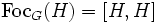# Subgroup whose focal subgroup equals its derived subgroup

This article defines a subgroup property: a property that can be evaluated to true/false given a group and a subgroup thereof, invariant under subgroup equivalence. View a complete list of subgroup properties[SHOW MORE]

## Definition

A subgroup$H$ of a group$G$ is termed a subgroup whose focal subgroup equals its commutator subgroup if we have the following condition. Let$\operatorname{Foc}_G(H)$ denote the focal subgroup of$H$ in$G$:$\operatorname{Foc}_G(H) = \langle xy^{-1} \mid x,y \in H, \exists g \in G, gxg^{-1} = y \rangle$.

Then, we require that:$\operatorname{Foc}_G(H) = [H,H]$,

i.e., the focal subgroup of$H$ equals its own commutator subgroup.